• 时间序列相似性度量综述

千次阅读 2021-02-03 03:53:08
时间序列相似性属于曲线相似性/曲线匹配(curve matching)领域的内容，在这一领域，有许多有用的方法，但是国内的博客上鲜有这方面的内容，因此我选取了几种常用的方法进行一下综述性的阐述。衡量相似性之前，我们...

时间序列相似性属于曲线相似性/曲线匹配(curve matching)领域的内容，在这一领域，有许多有用的方法，但是国内的博客上鲜有这方面的内容，因此我选取了几种常用的方法进行一下综述性的阐述。

衡量相似性之前，我们首先定义“相似”。

正常情况下，我们认为x,y,z是形状相似的，在这三条曲线中，我们认为y，z是最相似的两条曲线(因为y,z的距离最近)。

ok，那我们先来看看寻常意义上的相似：距离最近且形状相似。本文主要详细介绍时间序列相似度计算的DTW算法和PLR算法。

1. 欧式距离

要衡量距离与形状，显然欧式距离是一个天然完美的指标，上图中我们正是基于欧式距离认为y与z是最相似的，欧式距离在诸多算法都有广泛的应用。对于长度相同的序列，计算每两点之间的距离然后求和，距离越小相似度越高(whole matching)。对于不同长度的序列，一般有两种方法处理：

1)子序列匹配(subsequence matching): 找出长序列中与短序列最相似的部分。举个栗子，设序列,序列，其中。滚动地计算A与B的距离：，然后找出所有d中的最小值，该距离所对应的A序列的索引即为A中与B最相似的部分。

2)滑动窗口：微软在2001年在Dimensionality Reduction for Fast Similarity Search文中提出为了减少算法复杂度，可以复制B序直到与A序列等长。

由于微软之后使用了独特的降维方法，且计算复杂度不是本文考虑的主要内容，因此，在涉及长短序列相似度计算的时候，本文均使用第一种方法。

似乎时间序列的相似性度量的计算可以就此为止了，然而远非如此。

天津大学的XIAO-LI DONG, CHENG-KUI GU, ZHENG-OU WANG在2006年Research on shape-based time series similarity measure[C]//2006 International Conference on Machine Learning and Cybernetics. IEEE, 2006: 1253-1258一文中指出了欧式距离用于衡量时间序列相似性的三个缺陷：不能辨别形状相似性

不能反映趋势动态变化幅度的相似性

基于点距离的计算不能反映不同分析频率的不同

举个栗子：

A与B的变化趋势几乎完全相反，A与C的变化趋势几乎完全相同。如果使用欧式距离去度量，那么结论就是A与B是最相似的。而实际上，在变化是A与C是相似的。

为了进一步加强对欧式距离的理解，我们不妨再举一个简单的例子：

正常来说，我们认为与y1最相似的是y3，实际上，y3就是y1向下平移得到的。然而欧式距离告诉我们，距离y1最近的是y2。

下面是使用Python进行模拟的源代码：

import numpy as np

import matplotlib.pyplot as plt

x=np.arange(0,np.pi*2,0.1)

y1=np.sin(x)

y2=np.cos(x)-2

y3=y1-2

plt.plot(y1)

plt.plot(y2)

plt.plot(y3)

plt.legend(['y1','y2','y3'])

def dis(x,y):

return(sum(x-y)**2)

dis(y1,y2)

Out: 15831.914509398732

dis(y1,y3)

Out: 15876.0

欧式距离对形状的度量如此糟糕，有没有更好的模型能度量形状呢？

2. 模式距离Pattern distance

首先引入一个算法，PLR(piecewise linear representation)分段线性表示。

一个时间序列，无非有三种状态：上升、下降和不变，我们将这种状态对应表示为。假设有某个长度为S的序列，我们将其等分为K段。对于每一段计算一个斜率，斜率为正表示上升，为负表示下降，为0表示不变。

我画了一个草图，可能emm……不是很美观。

那么我们就可以将这个序列表示为[1,1,0,-1...]这样的序列，将相邻的相同模式进行合并，我们得到[1,0,-1...]的序列。这就是PLR算法。

关于PLR算法的点的分割，为了便于说理，我这里直接用的是等分的方式，然而观察上图可知，第三个模式表示为了0，实际上第三个模式是一个尖峰，是先上升后下降的，所以等分切割的方法并不科学。

KEOGH E. 在Fast similarity search in the presence of longitudinal scaling in time series data bases中提出的自底向上的搜索方法很好的解决了这个问题，感兴趣的读者可以自行了解。

因为我们将相邻的相同模式进行了合并，所以我们得到的模式序列一定是1,-1,0间隔排列的，每一个模式可能跨越了不同的时间长度，在合并模式后，序列S1可能有N个模式，S2可能有M个模式。现在我们要将他们等模式数化。

如上图所示，经过PLR后，我们将S1，S2表示为：分表表示S1,S2的第i,j个模式，即，t表示时间，且无论怎么切割，最后的终点都是相等的，即。结合上图，具体一点就是：等模式数化后将他们变形为：说白了就让他们使用共同的分割点，以获得最后长度相等的模式序列。

OK现在我们已经完全定义好了何为模式，接下来我们计算距离。这里我们使用绝对值距离，当然使用欧式距离也是可以的。模式距离公式为：，显然，距离越靠近0，表示模式越相似；越靠近2，表明模式越不相似。把所有的模式距离加起来即时间序列的模式距离：嗯……目前为止看起来还不错。但是需要注意的是，每一个模式可能跨越了不同的时间长度，而一个模式持续时间越长，它包含整个序列的信息就越多，这启发我们需要进行加权。因此：，其中为第i个模式所跨越的时间长度，为总时间长度。

3. 形状距离shape distance

在模式距离的基础上，XIAO-LI DONG, CHENG-KUI GU, ZHENG-OU WANG提出了形状距离，进一步提升了度量效果。该算法于2006年在国际机器学习与控制会议上提出。

如果理解了pattern distance的计算，那么理解shape distance将会非常简单，因为shape distance归根到底、总而言之就是加了一个振幅的改变量并重新设定了模式序列。

假设我们已经得到了等模式数化后的序列：设振幅amplitude改变量序列为A，则：，就是我们每一个分割区间的端点对应的序列值值差，那么我们可以得到：形状距离的最终计算公式为：，同样为时间权重。注意原序列S有N个点，模式序列和振幅改变量序列都是只有N-1个点。而这里的模式m也要重新定义。

下面以股票为例进行说明。我们认为股票的价格走势通常有七种状态：{加速下降，水平下降，减速下降，不变，减速上升，水平上升，加速上升}，我们用模式来描述这一点。

现在我们设定一个阈值th来帮助我们区分这7种状态，设Ki表示通过PLR划分后的第K段直线的斜率，还记得在模式距离中怎么设定模式的吗？

如果斜率小于0，则表示下降，记为-1；斜率大于0，则表示上升，记为1；如果斜率等于0，则表示不变，那么记为0。

现在情况稍微复杂了一些，因为我们引入了更多的模式(7种)。此时属于{-3，-2，-1}中的一种，那如何来知晓这支股票是加速下降、水平下降还是减速下降呢？斜率的改变量可以帮助我们，如果下一期斜率的改变量小于0，那说明斜率在递减，直线变得更陡峭了，是加速下降的，因此设为-3模式。如果，那么是水平下降的，设为-2。如果,说明是减速下降的，设为-1。如果斜率的在这个范围内，那么就认为是近似不变的，设为0。以此类推。

用一张表来总结一下：

形状距离公式是满足以下四个距离定理的：

此外，为了提高模型的准确度，较少绝对值的差异对整个模型的影响，一般需要对原序列进行标准化处理。完整的算法流程图如下：

形状距离由于加入了更多的模式，使得距离的度量更加精确，效果会好于模式距离。

4. DTW

讲了这么多距离，有没有更简单的方法来度量时间序列的相似性呢？相关系数可以吗？

相关系数是一个非常优美的指标，不仅能衡量相关性，也能衡量相似性，但是在时间序列中一般不使用相关系数衡量相似性，这是因为它不能解决图形平移的问题。

还是举个栗子~

y1与y2是平移的关系，显然与y1最相似的应该是y2，然而相关系数告诉我们是y3。

下面是Python进行模拟的代码：

import numpy as np

x=np.arange(-np.pi*2,np.pi*2,0.1)

y1=np.sin(x)

y2=np.cos(x)

y3=x

plt.plot(y1)

plt.plot(y2)

plt.plot(y3)

plt.legend(['y1','y2','y3'])

np.corrcoef([y1,y2,y3])

Out:

array([[ 1.00000000e+00, -1.76804105e-04, -3.88194383e-01],

[-1.76804105e-04, 1.00000000e+00, -1.28528471e-02],

[-3.88194383e-01, -1.28528471e-02, 1.00000000e+00]])

DTW(dynamic time warping)动态时间规整就是专为解决此问题而生~

DTW实际上是计算欧式距离，我们在之前讲过，欧式距离不能很好地度量形状相似性。左边这幅图更清楚地表现了这一点，按照一般欧式距离的计算方法，所有点直接对应下来。而DTW就是找到序列之间正确对应的点再计算他们的距离。而由于DTW这一出色的特质，这使得DTW在语音识别领域有着广泛的应用。因为一个音节，它可能拖的很长或很短，如何去正确地识别相似声音或音节对于语音识别至关重要。图源自：https://blog.csdn.net/zouxy09/article/details/9140207

如上图所示，a对应的点应该是b而不是b'，DTW的根本任务就是将点进行正确的对应。

那正确对应的标准是什么呢？

DTW认为，如果两个序列的点正确对应了，那么他们的距离(欧式距离)达到最小。

OK，现在问题很简单了，就找距离最小的点。一个序列的一个点可能对应另一个序列的多个点，如果穷举出所有的可能去找出最合适的点，这在时间复杂度上属于一个NP难的问题。我们需要一个行之有效的算法——动态规划。

由于DTW的理论比较晦涩，直接看公式让人云里雾里，为了更清楚简单地把DTW讲明白，我用Python进行一遍模拟的计算。

简单点，说话的方式简单点~~~

import pandas as pd

import numpy as np

import matplotlib.pyplot as plt

import seaborn as sns

x = np.array([1, 1, 2, 3, 2, 0])

y = np.array([0, 1, 1, 2, 3, 2, 1])

plt.plot(x,'r', label='x')

plt.plot(y, 'g', label='y')

plt.legend()

生成了两个长度不等的序列x,y。

distances = np.zeros((len(y), len(x)))

for i in range(len(y)):

for j in range(len(x)):

distances[i,j] = (x[j]-y[i])**2

distances

Out:

array([[1., 1., 4., 9., 4., 0.],

[0., 0., 1., 4., 1., 1.],

[0., 0., 1., 4., 1., 1.],

[1., 1., 0., 1., 0., 4.],

[4., 4., 1., 0., 1., 9.],

[1., 1., 0., 1., 0., 4.],

[0., 0., 1., 4., 1., 1.]])

计算两个序列的距离矩阵。横着表示x序列，竖着是y序列，比如说第0行第0个元素1表示x序列的第0个值和y序列的第0个值的距离(Python的索引从0开始)，即;再比如第4行第1列的元素4(如果你的第4行第1列元素没有找到4的话，请把索引从0开始)表示x序列第1个值和y序列的第4个值间的距离，即我们来做个可视化，颜色越深表示距离越远，比如说最远的距离是x的第三个值和y的第0个值之间的距离。

这里注意一下，我们的图形和距离矩阵是没有一一对应的。我们的距离矩阵中，索引0从左上角开始，图形从左下角开始。

def distance_cost_plot(distances):

plt.imshow(distances, interpolation='nearest', cmap='Reds')

plt.gca().invert_yaxis()#倒转y轴，让它与x轴的都从左下角开始

plt.xlabel("X")

plt.ylabel("Y")

# plt.grid()

plt.colorbar()

distance_cost_plot(distances)

现在我们计算一个累积距离矩阵，累积距离最小是我们的最终目标。

accumulated_cost = np.zeros((len(y), len(x)))

accumulated_cost[0,0] = distances[0,0]

accumulated_cost

Out:

array([[1., 0., 0., 0., 0., 0.],

[0., 0., 0., 0., 0., 0.],

[0., 0., 0., 0., 0., 0.],

[0., 0., 0., 0., 0., 0.],

[0., 0., 0., 0., 0., 0.],

[0., 0., 0., 0., 0., 0.],

[0., 0., 0., 0., 0., 0.]])

distance_cost_plot(accumulated_cost)

显然累积距离矩阵的第0行第0列=距离矩阵的第0行第0列=1，我们必须经过起点吧……如果我们一直往右走，那么累积距离距离矩阵：

for i in range(1, len(x)):

accumulated_cost[0,i] = distances[0,i] + accumulated_cost[0, i-1]

accumulated_cost

array([[ 1., 2., 6., 15., 19., 19.],

[ 0., 0., 0., 0., 0., 0.],

[ 0., 0., 0., 0., 0., 0.],

[ 0., 0., 0., 0., 0., 0.],

[ 0., 0., 0., 0., 0., 0.],

[ 0., 0., 0., 0., 0., 0.],

[ 0., 0., 0., 0., 0., 0.]])

distance_cost_plot(accumulated_cost)

如果我们一直往上走，那么：

for i in range(1, len(y)):

accumulated_cost[i,0] = distances[i, 0] + accumulated_cost[i-1, 0]

accumulated_cost

array([[ 1., 2., 6., 15., 19., 19.],

[ 1., 0., 0., 0., 0., 0.],

[ 1., 0., 0., 0., 0., 0.],

[ 2., 0., 0., 0., 0., 0.],

[ 6., 0., 0., 0., 0., 0.],

[ 7., 0., 0., 0., 0., 0.],

[ 7., 0., 0., 0., 0., 0.]])

distance_cost_plot(accumulated_cost)

好了，累积距离矩阵的计算方式已经完全明白了。在继续下去之前，必须讲明累积距离矩阵中路径的意义。我们的目标是找一条路径使得累积距离最小。从(0,0)开始，经过这个方块表示将x,y的第0个点对应起来，当然，起点是必须经过的~

如果路径经过(1,0)，表示将x的第1个点和y序列的第0个点对应起来。

如果路径经过(1,1)，表示将x的第1个点和y序列的第1个点对应起来。

所以，路径的前进方向只有三个：向右，向上，斜右上。

这是显然，我们的路径不能后退，如果路径向右或向上了多个方块，这表明一个序列的一个点对应了另一个序列的多个点。

目前为止，我们已经完成了累积距离矩阵的两列的计算。对于任何其他的点，累积距离的新增只可能来自于三个方向：左边，下边，斜左下。所谓动态规划就是我每前进一步都选取使我当前行进距离最小的方向。因此，对于任何其他的点，累积距离的计算公式为：现在，我们把累积距离矩阵计算完整：

for i in range(1, len(y)):

for j in range(1, len(x)):

accumulated_cost[i, j] = min(accumulated_cost[i-1, j-1], accumulated_cost[i-1, j], accumulated_cost[i, j-1]) + distances[i, j]

accumulated_cost

Out:

array([[ 1., 2., 6., 15., 19., 19.],

[ 1., 1., 2., 6., 7., 8.],

[ 1., 1., 2., 6., 7., 8.],

[ 2., 2., 1., 2., 2., 6.],

[ 6., 6., 2., 1., 2., 11.],

[ 7., 7., 2., 2., 1., 5.],

[ 7., 7., 3., 6., 2., 2.]])

distance_cost_plot(accumulated_cost)

现在，最佳路径已经清晰地显示在了累积距离矩阵之中，就是图中颜色最淡的方块。

现在，我们只需要通过回溯的方法找回最佳路径就可以了：

path = [[len(x)-1, len(y)-1]]

i = len(y)-1

j = len(x)-1

while i>0 and j>0:

if i==0:

j = j - 1

elif j==0:

i = i - 1

else:

if accumulated_cost[i-1, j] == min(accumulated_cost[i-1, j-1], accumulated_cost[i-1, j], accumulated_cost[i, j-1]):

i = i - 1#来自于左边

elif accumulated_cost[i, j-1] == min(accumulated_cost[i-1, j-1], accumulated_cost[i-1, j], accumulated_cost[i, j-1]):

j = j-1#来自于下边

else:

i = i - 1#来自于左下边

j= j- 1

path.append([j, i])

path.append([0,0])

path

Out: [[5, 6], [4, 5], [3, 4], [2, 3], [1, 2], [1, 1], [0, 1], [0, 0]]

path_x = [point for point in path]

path_y = [point for point in path]

distance_cost_plot(accumulated_cost)

plt.plot(path_x, path_y)

在刚才，我们已经讲明，最优路径所经过的地方表示两个序列应该对应的点。

计算这些点的欧式距离作为相似性的度量，这就是DTW。

Reference:Dong X L, Gu C K, Wang Z O. Research on shape-based time series similarity measure[C]//2006 International Conference on Machine Learning and Cybernetics. IEEE, 2006: 1253-1258.

Wang D, Rong G. Pattern distance of time series[J]. Zhejiang Daxue Xuebao(Gongxue Ban)/Journal of Zhejiang University(Engineering Science), 2004, 38(7): 795-798.

Kim S, Lee H, Ko H, et al. Pattern Matching Trading System Based on the Dynamic Time Warping Algorithm[J]. Sustainability, 2018, 10(12): 4641.

Keogh E, Chakrabarti K, Pazzani M, et al. Dimensionality reduction for fast similarity search in large time series databases[J]. Knowledge and information Systems, 2001, 3(3): 263-286.

Mori U, Mendiburu A, Lozano J A. Distance measures for time series in R: The TSdist package[J]. R journal, 2016, 8(2): 451-459.

展开全文• 时间序列相似性度量是时间序列相似性检索、时间序列无监督聚类、时间序列分类以及其他时间序列分析的基础。给定时间序列的模式表示之后，需要给出一有效度量来衡量两个时间序列的相似性。时间序列的相似性可以分为...

前言

时间序列相似性度量是时间序列相似性检索、时间序列无监督聚类、时间序列分类以及其他时间序列分析的基础。给定时间序列的模式表示之后，需要给出一个有效度量来衡量两个时间序列的相似性。时间序列的相似性可以分为如下三种：

1、 时序相似性

• 时序相似性是指时间序列点的增减变化模式相同，即在同一时间点增加或者减少，两个时间序列呈现一定程度的相互平行。这个一般使用闵可夫斯基距离即可进行相似性度量。

2、 形状相似性

• 形状相似性是指时间序列中具有共同的形状，它通常包含在不同时间点发生的共同的趋势形状或者数据中独立于时间点相同的子模式。两个时间序列整体上使用闵可夫斯基距离刻画可能不相似，但是他们具有共同相似的模式子序列，相似的模式子序列可能出现在不同的时间点。这个一般使用DTW动态时间规整距离来进行相似性刻画。

3、变化相似性

• 变化相似性指的是时间序列从一个时间点到下一个时间点的变化规律相同，两个时间序列在形状上可能并不一致，但是可能来自于同一个模型。这个一般使用ARMA或者HMM等模型匹配方法进行评估。

时间序列相似性度量可能会受到如下因素影响：

• 时间序列作为真实世界的系统输出或者测量结果，一般会夹杂着不同程度的 噪声扰动
• 时间序列一般会呈现各种变形，如
• 振幅平移
• 振幅压缩
• 时间轴伸缩
• 线性漂移
• 不连续点等
• 时间序列之间可能存在不同程度的关联；

以上因素在衡量时间序列相似性度量的时候要根据具体情况进行具体分析。

闵可夫斯基距离

给定两条时间序列：
P = ( x 1 , x 2 , . . . x n ) ,    Q ( y 1 , y 2 , . . . y n ) P=(x_1,x_2,...x_n),\ \ Q(y_1,y_2,...y_n)
闵可夫斯基距离的定义如下:
d i s t ( P , Q ) = ( ∑ i = 1 n ∣ x i − y i ∣ p ) 1 p dist(P,Q) = \left(\sum\limits_{i=1}^n|x_i-y_i|^p\right)^{\frac{1}{p}}
注：

1. p = 1 p=1 时，闵可夫斯基距离又称为 曼哈顿距离
d i s t ( P , Q ) = ∑ i = 1 n ∣ x i − y i ∣ dist(P,Q)=\sum\limits_{i=1}^n |x_i-y_i|

2. p = 2 p=2 时，闵可夫斯基距离又称为 欧氏距离
d i s t ( P , Q ) = ( ∑ i = 1 n ∣ x i − y i ∣ 2 ) 1 2 dist(P,Q) = \left(\sum\limits_{i=1}^n|x_i-y_i|^2\right)^{\frac{1}{2}}

3. p → ∞ p\rightarrow\infty 时，闵可夫斯基距离又称为 切比雪夫距离
lim ⁡ p → ∞ ( ∑ i = 1 n ∣ x i − y i ∣ p ) 1 p = max ⁡ i ∣ x i − y i ∣ \lim\limits_{p\rightarrow\infty}\left(\sum\limits_{i=1}^n|x_i-y_i|^p\right)^{\frac{1}{p}} = \max\limits_{i}|x_i-y_i|

4. 闵可夫斯基距离模型简单，运算速度快。

5. 闵可夫斯基距离比较直观，但是它与数据的分布无关，具有一定的局限性

• 在时间轴伸缩和弯曲情况下无法给出确切的度量

• 只能针对长度相等的时间序列

• 如果时间序列 P P 的幅值远远大于 Q Q 的幅值，那么得到的计算结果就会被时间序列 P P 所过度作用。

• 计算之前需要对数据进行尺度变化，例如减去均值并除以标准差，或者极大极小归一化

6. 这种方法在假设数据各个维度不相关的情况下，利用数据分布的特性计算出不同的距离，如果数据维度之间数据相关，这时该类距离就不合适了！

余弦相似度

给定两个时间序列：
P = ( x 1 , x 2 , . . . x n ) ,    Q ( y 1 , y 2 , . . . y n ) P=(x_1,x_2,...x_n),\ \ Q(y_1,y_2,...y_n)
余弦相似度如下：
D c o s ( P , Q ) = ∑ i = 1 n x i y i ∑ i = 1 n x i 2 ∑ i = 1 n y i 2 D_{cos}(P,Q) = \frac{\sum\limits_{i=1}^n x_iy_i}{\sqrt{\sum\limits_{i=1}^nx_i^2}\sqrt{\sum\limits_{i=1}^ny_i^2}}

动态时间规整距离

动态时间规整距离是一种动态规划方法，通过寻找最小的对齐匹配路径使两序列之间距离最小，因而DTW可计算不同长度序列的距离，对时间序列偏移不敏感。

给定两个时间序列（两个时间序列并一定等长，即m和n不一定相等）：
P = ( x 1 , x 2 , . . . x m ) ,    Q ( y 1 , y 2 , . . . y n ) P=(x_1,x_2,...x_m),\ \ Q(y_1,y_2,...y_n)
A m × n = ( a i j ) m × n A_{m\times n}=(a_{ij})_{m\times n} 表示 P P Q Q 的距离矩阵，其中 a i j a_{ij} 基于基距离方法进行计算，一般采用欧氏距离的平方：
a i j = ( x i − y j ) 2 a_{ij} = (x_i-y_j)^2
然后在距离矩阵中，令 W = w 1 , w 2 , . . . , w k W=w_1,w_2,...,w_k 为时间序列 P P Q Q 的动态时间弯曲路径，其中 w k = ( a i j ) k w_k=(a_{ij})_k 是路径的第k个元素，且路径 W W 需要满足以下条件：

1、 max ⁡ { m , n } ≤ K ≤ m + n − 1 \max\{m,n\}\leq K\leq m+n-1

2、 w 1 = a 11 , w k = a m n w_1=a_{11}, w_k=a_{mn}

3、单调性：若 w k = a i j , w k + 1 = a k l w_k=a_{ij}, w_{k+1}=a_{kl} ，则需保证
0 ≤ k − i ≤ 1 ,    0 ≤ l − j ≤ 1 0\leq k-i\leq 1, \ \ 0\leq l-j\leq 1
根据上述三个条件可知，动态时间弯曲路径并不唯一，在所有的动态时间弯曲路径中使得 ∑ i = 1 K w i \sqrt{\sum\limits_{i=1}^K w_i} 的值最小的路径称为最佳动态时间弯曲路径，所对应的距离即为动态时间弯曲距离。记 D T W ( P , Q ) DTW (P,Q) 为P和Q之间的DTW距离，则有：
D T W ( P , Q ) = min ⁡ ( ∑ i = 1 K w i ) DTW(P,Q) = \min\left(\sqrt{\sum\limits_{i=1}^{K}w_i}\right)
为什么称DTW为动态时间规整距离呢？

因为DTW距离的计算过程是一个 动态规划过程， 若令 P P Q Q i i j j 处累积距离为 L ( i , j ) L(i,j) ，则由条件3可知，累积距离存在如下递归关系：
L ( i , j ) = min ⁡ [ L ( i − 1 , j − 1 ) , L ( i − 1 , j ) , L ( i , j − 1 ) ] + a i j L(i,j) = \min\left[L(i-1,j-1), L(i-1,j), L(i,j-1)\right]+a_{ij}
易知： L ( 1 , 1 ) = a 11 L(1,1)=a_{11} ， 且 D T W ( P , Q ) = L ( m , n ) DTW(P,Q)=L(m,n) ，因此DTW的计算过程就是：

• 先使用基距离，如欧式距离初始化距离矩阵 A m × n = ( a i j ) m × n A_{m\times n}=(a_{ij})_{m\times n}
• 然后使用累积距离的递归公式求解得到 L ( m , n ) L(m,n) 即为DTW距离

注：

1. DTW距离通过允许序列在时间轴上偏移，克服了闵可夫斯基距离不能度量不等长序列、对于噪声和振幅伸缩无法刻画的缺陷
2. 可以度量任意两个长度时间序列间的距离，对时间轴上的扭曲不敏感，适用性、可靠性更强
3. 计算复杂度高： O ( m n ) O(mn) ， 时间代价昂贵
4. DTW距离不满足三角不等式
5. 相应改进方案：下界函数、加权动态时间规整距离、微分动态时间规整距离、FastDTW

基于相关性的相似度度量方法

给定两个时间序列：
P = ( x 1 , x 2 , . . . x n ) ,    Q ( y 1 , y 2 , . . . y n ) P=(x_1,x_2,...x_n),\ \ Q(y_1,y_2,...y_n)
相关系数定义如下：
C o r ( P , Q ) = C o v ( P , Q ) V a r ( P ) V a r ( Q ) Cor(P,Q) = \frac{Cov(P,Q)}{\sqrt{Var(P)\sqrt{Var(Q)}}}
相关系数为1，表示完全一致；相关系数为-1，表示完全负相关；相关系数为0，表示没有相关性

基于互信息的相似性度量方法

给定两个时间序列：
P = ( x 1 , x 2 , . . . x n ) ,    Q ( y 1 , y 2 , . . . y n ) P=(x_1,x_2,...x_n),\ \ Q(y_1,y_2,...y_n)
互信息定义如下：
I ( P , Q ) = H ( P ) + H ( Q ) − H ( P , Q ) I(P,Q)=H(P)+H(Q)-H(P,Q)
互信息取值范围为 [ 0 , ∞ ] [0,\infty] ，互信息为0表示两者之间没有公共信息，互信息为 ∞ \infty 表示相关性特别大，类似于相关系数的绝对值为1. 除此之外，可利用如下公式对互信息进行归一化：
Λ ( P , Q ) = 1 − e 2 I ( P , Q ) \Lambda(P,Q) = \sqrt{1-e^{2I(P,Q)}}

基于互信息的相似性度量方法（可能拓展）

上面基于互信息的相似性度量并没有考虑到不同时间序列之间的时间延迟关系，如果他们存在一定程度的时间延迟，那么可以考虑如下方式的拓展：

• 计算延迟互信息，然后对不同的时间延迟所得互信息求最大值或者直接求和
• 计算转移熵，然后求最大值或者直接求和

马氏距离

上面提到了基于相关性和基于互信息的距离，仅仅考虑到了变量之间的相关性，而马氏距离则是同时考虑到变量不同维度之间存在相关性和尺度变换的关系，它表示的是变量之间的协方差距离。

假设有两个n维空间中的点：
P = ( x 1 , x 2 , . . . x n ) ,    Q ( y 1 , y 2 , . . . y n ) P=(x_1,x_2,...x_n),\ \ Q(y_1,y_2,...y_n)
那么马氏距离的定义为：
D M ( P , Q ) = ( P − Q ) T Σ − 1 ( P − Q ) D_M(P,Q) = \sqrt{(P-Q)^T\Sigma^{-1}(P-Q)}
其中 Σ \Sigma 是个协方差矩阵，但是貌似跟 P P Q Q 没啥关系，它应该是个 n × n n\times n 的矩阵， 刻画了n个属性之间的关系，计算方式如下：

• 对n维变量进行采样，采样得到样本集合： { P 1 , P 2 , . . . , P m } \{P_1,P_2,...,P_m\} ，这个集合的规模可能比 n n 大很多， 然后每个属性有一个时间序列，计算各个属性之间的协方差矩阵，以及各个属性的均值组成的均值向量 μ = [ μ 1 , μ 2 , . . . , μ n ] \mu=[\mu_1,\mu_2,...,\mu_n]

如果利用矩阵分解，如LU分解对协方差矩阵 Σ \Sigma 进行分解的话： Σ = L L T \Sigma=LL^T ，然后对 P − Q P-Q 做如下处理：
Z = L − 1 ( P − Q ) Z = L^{-1}(P-Q)
则马氏距离可以表示为：
D M ( P , Q ) = Z T Z D_M(P,Q) = Z^TZ
特别地，马氏距离可以用来衡量一个点 P 和样本集合 { P 1 , P 2 , . . . , P m } \{P_1,P_2,...,P_m\} 之间的距离，这是通过比较 P 和均值向量 μ \mu 得到的：
D M ( P ) = ( P − μ ) Σ − 1 ( P − μ ) D_M(P) = \sqrt{(P-\mu)\Sigma^{-1}(P-\mu)}
注：

• 马氏距离计算的不是时间序列之间的距离，而是对欧氏距离的推广，计算的是两个点之间的距离
• 马氏距离需要一个样本集合来估算样本均值和协方差。
• 马氏距离相当于将变量按照主成分进行旋转，让维度之间相互独立，然后进行标准化，让维度同分布
• 主成分就是特征向量方向，每个方向的方差就是对应的特征值，所以其实就是按照特征向量的方向进行旋转，然后缩放特征值倍

优点：

• 马氏距离不受量纲的影响，且由标准化数据和中心化数据计算出的两点之间的马氏距离相同
• 马氏距离可以排除变量之间相关性的干扰

缺点：

• 需要计算协方差矩阵，协方差矩阵还需要可逆
• 涉及到矩阵求逆
• 不能处理非线性流形上的问题

参考文献

时间序列聚类相关知识的总结与梳理: https://mp.weixin.qq.com/s/iusTb9UwKybwJBqd2kSvUA

机器学习基础 | 互相关系数和互信息异同探讨：https://www.cnblogs.com/fangsf/p/15000465.html

荣馨兵. 基于相似搜索的时间序列预测方法及其应用[D]. 大连海事大学, 2018.

张勇. 时间序列模式匹配技术研究[D]. 华中科技大学. 2012

展开全文• 时间序列相似性的度量方法可分为三类: (1)基于时间步长的，如反映逐点时间相似性的欧氏距离； (2)基于形状，如Dymanic Time Warping(Berndt和Clifford 1994)根据趋势出现； (3)基于变化的，如高斯混合模型(GMM) ...

参考链接：
https://blog.csdn.net/xsdxs/article/details/86648605
https://zhuanlan.zhihu.com/p/117634492
https://blog.csdn.net/u010194274/article/details/52183453
论文中应用：

时间序列相似性的度量方法可分为三类:
(1)基于时间步长的，如反映逐点时间相似性的欧氏距离；
(2)基于形状，如Dymanic Time Warping(Berndt和Clifford 1994)根据趋势出现；
(3)基于变化的，如高斯混合模型(GMM) (Povinelli等人，2004)，它反映了数据生成过程的相似性。

1. 欧氏距离与DTW

描述两个序列之间的相似性，欧氏距离是一种十分简单且直观的方法，但对于序列之间out of phase的情况，计算欧氏距离得到的结果会比实际的最小距离大很多，比如下面两个几乎一样的序列:左边是欧氏距离的对应关系，在同一时刻上的点相互对应，计算总距离；右边是DTW的点对应关系，可以看到，下面序列某时刻的点可以对应上面序列非同一时刻的点，同时一个点可以对应多个点，多个点也可以对应一个点，也就是说每个点尽可能找离它距离最小的点，允许时间轴上的伸缩。显而易见的，这种情况下DTW计算的距离比欧氏距离更小。因此对于时间上有拉伸或压缩的序列，使用DTW计算的序列距离更加合理，因此该算法在语音序列匹配中使用十分广泛。

2.DTW的思想

见图：我们有两个序列C和Q， C n = { c 1 , c 2 , . . . , c n } , Q m { q 1 , q 2 , . . . , q m } C_n=\{c_1,c_2,...,c_n\},Q_m\{q_1,q_2,...,q_m\} ，要计算两者之间的距离，先画一个 m × n m \times n 的二维数组，数组中的每个点 w ( i , j ) w(i,j) 代表 Q i Q_i C j C_j 的距离 ( Q i − C j ) 2 \sqrt{(Q_i-C_j)^2} ，DTW的核心思想是在这样的一个距离矩阵中从两个序列的起点找到通往两个序列终点（即对角线的一端到另一端）的最小距离路径（如图B中灰色方块，可通过动态规划求解，下文有具体例子介绍），但是在寻找路径的过程中，必须满足一些约束条件：

1、边界条件：起点必须是 w ( 1 , 1 ) w(1,1) ，终点必须是 w ( m , n ) w(m,n) ，要有始有终；
2、连续性：意思是下一个满足条件的灰色方块一定是在当前灰色方块的周围一圈；
3、单调性：下一个满足条件的灰色方块一定在当前灰色方块的右上方，不能回头；

满足2、3条件的最终搜索方向如下3.DTW计算序列距离——举例说明

假设有两个序列P=[1,3,2,4,2]，Q=[0,3,4,2,2]，直接计算两者的欧氏距离为5（这里直接用差值代替平方项）：如何使用动态规划通过DTW求最小距离呢？

首先计算两个序列的距离矩阵：从左上角开始, 可以向右,向下,或者向右下前进, 对进行到这三个方向后的距离累加和进行比较, 易知向右累加距离和为3, 向下为4, 向右下为1, 因此选择向右下前进. 如果用dp(i,j)表示二维矩阵中第(i,j)位置的最小距离, 那么状态转移方程可以表示为:

d p ( i , j ) = min ⁡ ( d p ( i − 1 , j − 1 ) , d p ( i − 1 , j ) , d p ( i , j − 1 ) ) + d ( i , j ) dp(i,j)=\min(dp(i-1,j-1),dp(i-1,j),dp(i,j-1))+d(i,j)
其中 d ( i , j ) d(i,j) P i P_i Q j Q_j 的距离。
以此类推, 最终构建的距离累加和矩阵为:最优路径如表中灰色方块所示, 最终得到的最短距离为矩阵右下角最后一个数2, 比起欧式距离的5小很多. 两个序列的其对应关系如下:python代码示例如下：

# 计算序列组成单元之间的距离，可以是欧氏距离，也可以是任何其他定义的距离,这里使用绝对值
def distance(w1,w2):
d = abs(w2 - w1)
return d

# DTW计算序列s1,s2的最小距离
def DTW(s1,s2):
m = len(s1)
n = len(s2)

# 构建二位dp矩阵,存储对应每个子问题的最小距离
dp = [*n for _ in range(m)]

# 起始条件,计算单个字符与一个序列的距离
for i in range(m):
dp[i] = distance(s1[i],s2)
for j in range(n):
dp[j] = distance(s1,s2[j])

# 利用递推公式,计算每个子问题的最小距离,矩阵最右下角的元素即位最终两个序列的最小值
for i in range(1,m):
for j in range(1,n):
dp[i][j] = min(dp[i-1][j-1],dp[i-1][j],dp[i][j-1]) + distance(s1[i],s2[j])

return dp[-1][-1]

s1 = [1,3,2,4,2]
s2 = [0,3,4,2,2]

print('DTW distance: ',DTW(s1,s2))   # 输出 DTW distance:  2

DTW的修改

4.修改背景

最近项目中遇到求解时间序列相似性问题，这里序列也可以看成向量。在传统算法中，可以用余弦相似度和pearson相关系数来描述两个序列的相似度。但是时间序列比较特殊，可能存在两个问题：

两段时间序列长度不同。如何求相似度？
一个序列是另一个序列平移之后得到的。如何求相似距离？

第一个问题，导致了根本不能用余弦相似度和pearson相关系数来求解相似。第二个问题，导致了也不能基于欧式距离这样的算法，来求解相似距离。比如下面两个长度不同的序列：

s1 = [1, 2, 0, 1, 1, 2]
s2 = [1, 0, 1]

本文记录一种算法，一方面：如果两个序列中的其中一个序列是另一个序列的平移序列，则可以比较合理的求出两个序列的相似距离。另一方面：也可以求解长度不同序列的相似距离。

同时基于这个算法，先计算相似距离，再把相似距离通过 1 1 + d i s t \frac{1}{1+dist}
转化为相似度。这样就可以得到长度不同向量的相似度.

5.核心思想

Dynamic Time Warping (DTW) 本质上和通过动态规划来计算这个序列的相似距离。其实这和求解字符串的最长公共子串、子序列这类问题本质比较类似。可以参考

• 两个字符串的最长子串
• 两个字符串的最长子序列

6.应用的问题

其实在实际使用中，我们发现该算法对周期序列的距离计算不是很好。尤其两个序列是相同周期，但是是平移后的序列。如下：

s1 = np.array([1, 2, 0, 1, 1, 2, 0, 1, 1, 2, 0, 1, 1, 2, 0, 1])
s2 = np.array([0, 1, 1, 2, 0, 1, 1, 2, 0, 1, 1, 2, 0, 1, 1, 2])
s3 = np.array([0.8, 1.5, 0, 1.2, 0, 0, 0.6, 1, 1.2, 0, 0, 1, 0.2, 2.4, 0.5, 0.4])

用图表展示:很明显从图中可以看到$_1 和 和 s_2 是 相 同 的 时 间 序 列 ， 但 是 是相同的时间序列，但是 s_1 是 是 s_2$ 平移后得到的， s 3 s_3 是随意构造的序列。利用DTW求解时，得
d i s t ( s 1 , s 2 ) = 2.0 dist(s_1,s_2)=2.0
d i s t ( s 1 , s 3 ) = 1.794435844492636 dist(s_1,s_3)=1.794435844492636
发现 s 1 s_1 s 3 s_3 较为相像。因为需要对算法进行该井。

7.改进策略

7.1 改进策略1

目的是想求得一个惩罚系数 α \alpha ，这个 α \alpha 和原算法的distance相乘，得到更新后的distance.
首先，基于原算法求得 d p ( i , j ) dp(i,j) ,找到从左上角到右下角得最优路径。如图表示：
s 1 s_1 s 2 s_2 得路径：s 1 s_1 s 3 s_3 得路径：比较
s 1 s_1 s 2 s_2 得最优路径得拐点比较少，对角巷很长。而 s 1 s_1 s 3 s_3 拐点较多，对角线很短。原作者基于此考虑进行优化，公式如下：
α = 1 − ∑ i = 1 n c o m L e m i 2 s e q L e n 2 \alpha =1-\sqrt{\sum^n_{i=1}\frac{comLem^2_i}{seqLen^2}}
其中seLen是这个图中最优路径节点得个数， c o m L e n i comLen_i 表示每段对角直线得长度。求和后开发表示一个长度系数，这个长度系数越大，表示对角直线越长。最后1减去这个长度系数得到得衰减系数 α \alpha
代码实现：

import numpy as np
import math

def get_common_seq(best_path, threshold=1):
com_ls = []
pre = best_path
length = 1
for i, element in enumerate(best_path):
if i == 0:
continue
cur = best_path[i]
if cur == pre + 1 and cur == pre + 1:
length = length + 1
else:
com_ls.append(length)
length = 1
pre = cur
com_ls.append(length)
return list(filter(lambda num: True if threshold < num else False, com_ls))

def calculate_attenuate_weight(seqLen, com_ls):
weight = 0
for comlen in com_ls:
weight = weight + (comlen * comlen) / (seqLen * seqLen)
return 1 - math.sqrt(weight)

def best_path(paths):
"""Compute the optimal path from the nxm warping paths matrix."""
i, j = int(paths.shape - 1), int(paths.shape - 1)
p = []
if paths[i, j] != -1:
p.append((i - 1, j - 1))
while i > 0 and j > 0:
c = np.argmin([paths[i - 1, j - 1], paths[i - 1, j], paths[i, j - 1]])
if c == 0:
i, j = i - 1, j - 1
elif c == 1:
i = i - 1
elif c == 2:
j = j - 1
if paths[i, j] != -1:
p.append((i - 1, j - 1))
p.pop()
p.reverse()
return p

def TimeSeriesSimilarity(s1, s2):
l1 = len(s1)
l2 = len(s2)
paths = np.full((l1 + 1, l2 + 1), np.inf)  # 全部赋予无穷大
paths[0, 0] = 0
for i in range(l1):
for j in range(l2):
d = s1[i] - s2[j]
cost = d ** 2
paths[i + 1, j + 1] = cost + min(paths[i, j + 1], paths[i + 1, j], paths[i, j])

paths = np.sqrt(paths)
s = paths[l1, l2]
return s, paths.T

if __name__ == '__main__':
# 测试数据
s1 = np.array([1, 2, 0, 1, 1, 2, 0, 1, 1, 2, 0, 1, 1, 2, 0, 1])
s2 = np.array([0, 1, 1, 2, 0, 1, 1, 2, 0, 1, 1, 2, 0, 1, 1, 2])
s3 = np.array([0.8, 1.5, 0, 1.2, 0, 0, 0.6, 1, 1.2, 0, 0, 1, 0.2, 2.4, 0.5, 0.4])

# 原始算法
distance12, paths12 = TimeSeriesSimilarity(s1, s2)
distance13, paths13 = TimeSeriesSimilarity(s1, s3)

print("更新前s1和s2距离：" + str(distance12))
print("更新前s1和s3距离：" + str(distance13))

best_path12 = best_path(paths12)
best_path13 = best_path(paths13)

# 衰减系数
com_ls1 = get_common_seq(best_path12)
com_ls2 = get_common_seq(best_path13)

# print(len(best_path12), com_ls1)
# print(len(best_path13), com_ls2)
weight12 = calculate_attenuate_weight(len(best_path12), com_ls1)
weight13 = calculate_attenuate_weight(len(best_path13), com_ls2)

# 更新距离
print("更新后s1和s2距离：" + str(distance12 * weight12))
print("更新后s1和s3距离：" + str(distance13 * weight13))

输出：

更新前s1和s2距离：2.0
更新前s1和s3距离：1.794435844492636
更新后s1和s2距离：0.6256314581274465
更新后s1和s3距离：0.897217922246318

结论：
用新的算法更新后，我们会发现s1和s2距离比s1和s3的距离更加接近了，这就是我们要的结果。

7.2 改进策略2

原作者想也求得一个惩罚系数，方案如下：

1. 先求两个序列seq1和seq2得最长公共子串，长度记为a.
2. 因为seq1和seq2是数值序列，在求最长公共子串时，设置了一个最大标准差得偏移容忍.也就是说，两个数值在这个标准差内，认为也是公共子串得一部分。
3. 衰减系数：KaTeX parse error: Undefined control sequence: \timeslen at position 36: …es a}{len(seq1)\̲t̲i̲m̲e̲s̲l̲e̲n̲(seq2)}
也就是说，两个数值序列的最长公共子串越长，则衰减系数越小。这里把：s2 = np.array([0, 1, 1, 2, 0, 1, 1, 2, 0, 1, 1, 2, 0, 1, 1, 2])改成s2 = np.array([0, 1, 1, 2, 0, 1, 1.7, 2, 0, 1, 1, 2, 0, 1, 1, 2])，来验证最大标准差的偏移容忍。
代码和效果如下：
import numpy as np

float_formatter = lambda x: "%.2f" % x
np.set_printoptions(formatter={'float_kind': float_formatter})

def TimeSeriesSimilarityImprove(s1, s2):
# 取较大的标准差
sdt = np.std(s1, ddof=1) if np.std(s1, ddof=1) > np.std(s2, ddof=1) else np.std(s2, ddof=1)
# print("两个序列最大标准差:" + str(sdt))
l1 = len(s1)
l2 = len(s2)
paths = np.full((l1 + 1, l2 + 1), np.inf)  # 全部赋予无穷大
sub_matrix = np.full((l1, l2), 0)  # 全部赋予0
max_sub_len = 0

paths[0, 0] = 0
for i in range(l1):
for j in range(l2):
d = s1[i] - s2[j]
cost = d ** 2
paths[i + 1, j + 1] = cost + min(paths[i, j + 1], paths[i + 1, j], paths[i, j])
if np.abs(s1[i] - s2[j]) < sdt:
if i == 0 or j == 0:
sub_matrix[i][j] = 1
else:
sub_matrix[i][j] = sub_matrix[i - 1][j - 1] + 1
max_sub_len = sub_matrix[i][j] if sub_matrix[i][j] > max_sub_len else max_sub_len

paths = np.sqrt(paths)
s = paths[l1, l2]
return s, paths.T, [max_sub_len]

def calculate_attenuate_weight(seqLen1, seqLen2, com_ls):
weight = 0
for comlen in com_ls:
weight = weight + comlen / seqLen1 * comlen / seqLen2
return 1 - weight

if __name__ == '__main__':
# 测试数据
s1 = np.array([1, 2, 0, 1, 1, 2, 0, 1, 1, 2, 0, 1, 1, 2, 0, 1])
s2 = np.array([0, 1, 1, 2, 0, 1, 1.7, 2, 0, 1, 1, 2, 0, 1, 1, 2])
s3 = np.array([0.8, 1.5, 0, 1.2, 0, 0, 0.6, 1, 1.2, 0, 0, 1, 0.2, 2.4, 0.5, 0.4])

# 原始算法
distance12, paths12, max_sub12 = TimeSeriesSimilarityImprove(s1, s2)
distance13, paths13, max_sub13 = TimeSeriesSimilarityImprove(s1, s3)

print("更新前s1和s2距离：" + str(distance12))
print("更新前s1和s3距离：" + str(distance13))

# 衰减系数
weight12 = calculate_attenuate_weight(len(s1), len(s2), max_sub12)
weight13 = calculate_attenuate_weight(len(s1), len(s3), max_sub13)

# 更新距离
print("更新后s1和s2距离：" + str(distance12 * weight12))
print("更新后s1和s3距离：" + str(distance13 * weight13))

输出：

更新前s1和s2距离：2.0223748416156684
更新前s1和s3距离：1.794435844492636
更新后s1和s2距离：0.47399410350367227
更新后s1和s3距离：1.6822836042118463

8. 论文中得DTW

《Spatial-Temporal Fusion Graph Neural Networks for Traffic Flow Forecastin》

1. 给定两个不等时长时间序列：
X = ( x 1 , x 2 , . . . , x n ) X=(x_1,x_2,...,x_n) Y = ( y 1 , y 2 , . . . , y m ) Y=(y_1,y_2,...,y_m)
2. 构建序列距离矩阵(distance matrix) M n × m M_{n\times m} ,其元素是 M i , j = ∣ x i − y j ∣ M_{i,j}=|x_i-y_j|
3. 定义成本矩阵(cost matrix) M c M_c
M c ( i , j ) = M i , j + m i n ( M c ( i , j − 1 ) , M c ( i − 1 , j ) , M c ( i , j ) (1) M_c(i,j)=M_{i,j}+min(M_c(i,j-1),M_c(i-1,j),M_c(i,j)\tag{1}
4. 经过i和j得几次迭代，在X和Y直接按得最终距离也是最佳对齐表示为\$dist(X,Y),可以用来表示序列得相似度。

表明：ＤＷＴ是一种基于动态规划得算法，其核心是秋节扭曲曲线，即序列点 x i x_i y j y_j 之间得匹配。换句话说。"wraping path“ Ω \Omega 是由公式(1)生成得，
Ω = ( w 1 , w 2 , . . w λ ) , max ⁡ ( n , m ) ≤ λ ≤ n + m \Omega=(w_1,w_2,..w_{\lambda}), \max(n,m)\leq \lambda \leq n+m
它的元素 ω λ = ( i , j ) \omega_{\lambda}=(i,j) ,意思是 x i x_i y j y_j 搭配。

展开全文• 什么是DTW？ DTW算法采用了动态规划DP（dynamic programming）的方法来进行时间规整的计算，可以说，动态规划方法在时间规整问题上的应用就是DTW。 为什么需要DTW算法 ...如图所示，这两个序列整体上波形很相似

什么是DTW？

DTW算法采用了动态规划DP（dynamic programming）的方法来进行时间规整的计算，可以说，动态规划方法在时间规整问题上的应用就是DTW。

为什么需要DTW算法

当两个序列按照时间步t完全对齐的时候，我们可以直接使用ED算法（或者其它距离计算）来评估两个算法的相似度。但是有些时候两个序列并未完全对其，如果我们将某一序列进行压缩处理，此时会有信息损失。那么是否可以将两个长度不一样的序列进行对齐，然后再进行距离计算，DTW算法可以完成这个任务。

如图所示，这两个序列整体上波形很相似，但是在时间轴上确实对不齐的，所以这样如果按照时间步t对应来求距离显然会出问题。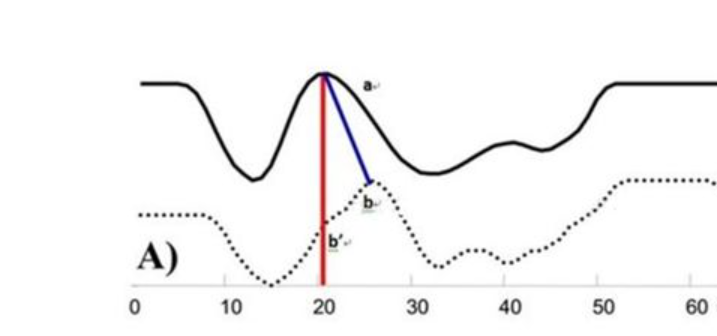为了解决这个问题，我们需要进行对齐操作：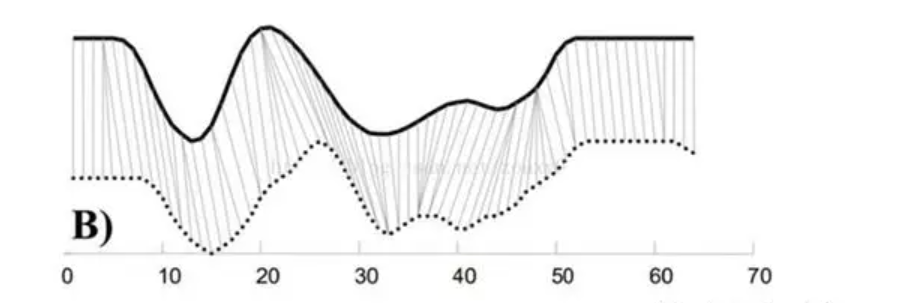如图所示，就是应用DTW算法之后的对齐之后的效果图，那么此时我们对两个序列的对应点之间计算距离，此时才是这两个序列的真实距离。

DTW的核心问题？

DTW核心是将两个不同的序列按照最好的方式对齐，而如何才是最好对齐呢？对齐的方式有很多，最好的对齐方式就是两个序列的距离最小，同时这个最小的距离就是这两个序列的距离。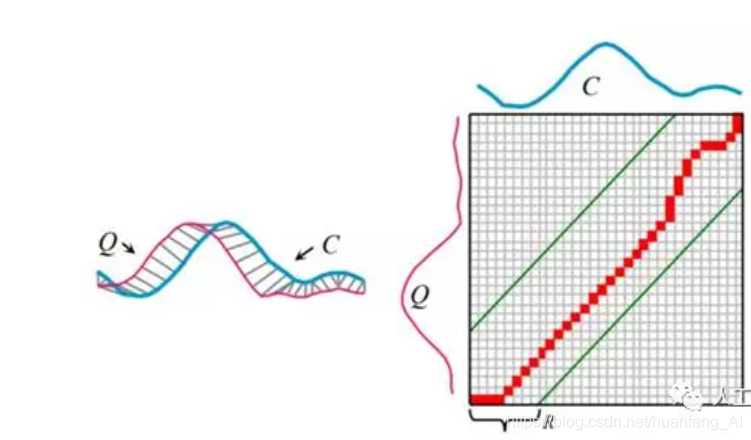如图所示，两条完全不同的序列Q，C，如何才能对齐呢？

假设Q的序列长度为n，而C的序列长度为m，那么我们需要构建一个n*m的矩阵，其中矩阵元素（i，j）表示Qi和Cj之间的距离。每个矩阵元素表示Qi和Cj对齐，那么从矩阵左下角到右上角可以找到很多路径（为什么是从左下角到右上角，因为两个不同的序列无论长短，它们的起始点和终止点肯定是对应的），这个矩阵包含了所有的对齐路径，DP算法就是要找到一条最短的路径。

路径的性质

首先这条路径需要满足一定的性质，它可以帮助算法对路径进行规范。

1. 边界条件：必须从矩阵的左上角到矩阵的右上角

2. 连续性：路径需要是连续的，不能跨越某点去匹配，（跨越其实也行，这样会有信息损失）

3. 单调性：路径必须随着时间单调进行，这样路径不会出现相交的情况。

连续性和单调性决定了路径中每一个格点只有三个方向，如果当前格点为（i，j），那么下一个格点只能是下面的三种情况（i+1，j）、（i，j+1）、（i+1，j+1）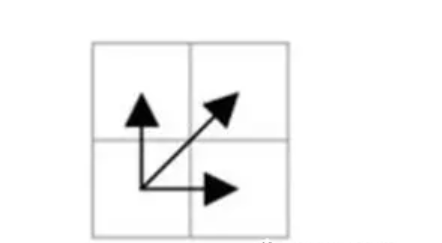当然如果连续性中考虑跨越的情况，那么可能来自五个方向，此时从(m-2,n-1)到（m，n）会有信息损失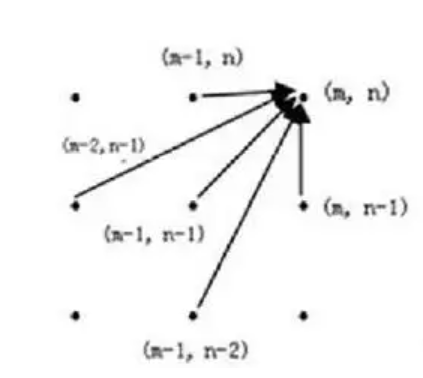本文中我们只考虑三种方向的情况。

动态规划关系表达式

现在我们已经知道了路径的规范条件了，那么这个问题的动态规划关系表达式为：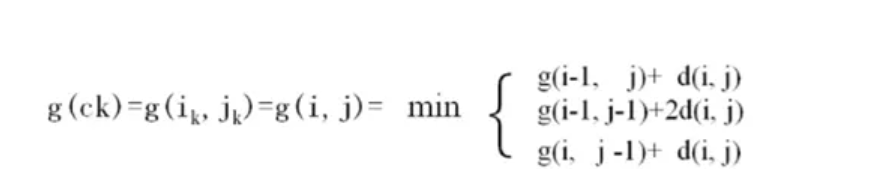我们拿g(i,j)=g(i-1,j)+d(i,j)来举例，g(i-1,j)我们可以认为是起点到g(i-1,j)的最短距离，然后d(i,j)表示Qi与Cj之间的距离。

为什么有d还有2d呢？

这个可以理解为人为设定的，我们这里设定当路径横着走还有竖着走的时候，就是d，当路径斜着走的时候就是2d，也就是此时我们设定斜着走的时候损失大一些，d可以认为是损失。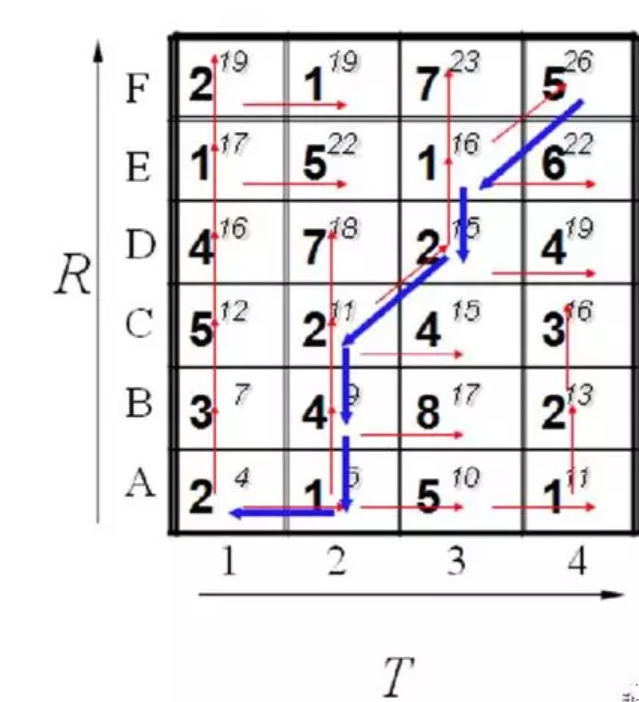现在有两个序列R和T，现在我们构建了一个矩阵，我们要找到从左下角到右上角的最短路径，每个格子中数字表示Ri和Tj的距离，右上角表示总距离。我们来看一下B2是如何计算的：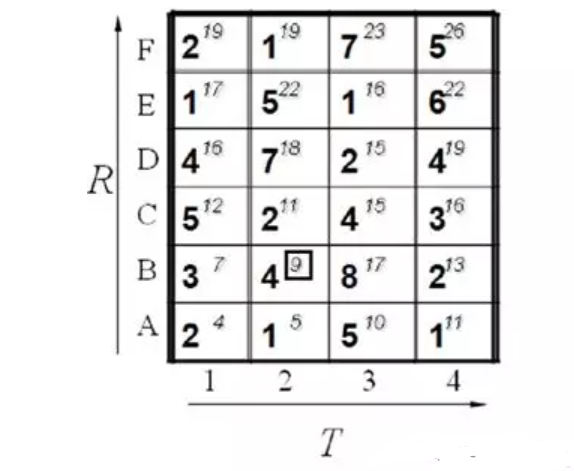如果只能从三个方向来走的话，B2可以认为来自B1、A1、A2，通过上面的动态规划关系式可以算出来

A1+2B2=4+24=12

B1+B2=7+4=11

A2+B2=5+4=9

所以从起点到B2的最短距离就是9，我们可以通过这种方式计算出格子的所有的点，那么最终我们可以算出从起点到F4的最短距离就是26，这就是动态规划，而动态规划在时间序列的应用就是DTW算法。

但是这里就有一个问题了？以上仅仅比较两条路径就要计算这么多，如果多条路径C与Q进行匹配，那么这个计算量就太大了，也就是说时间复杂度太高了，需要进行算法的改进。所以人们在使用DTW算法的时候就会使用一些技巧，以次来提高计算速度。

一些技巧

去根号计算

当使用DTW算法的时候，需要计算Q与C之间不同i，j之间的距离，那么往往需要较大的计算量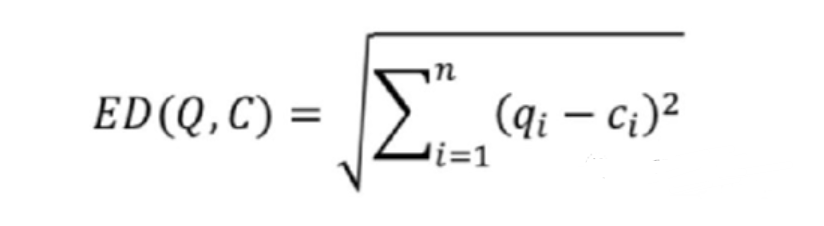我们可以看到我们的目的是为了寻找最小的计算量，而去掉根号不影响其大小的比较，而根号的计算需要耗费较多时间，所以一个技巧就是去掉根号。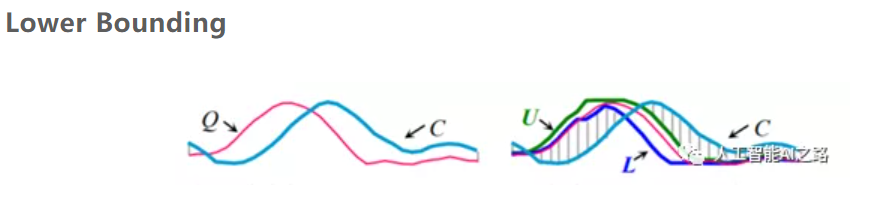Lower Bounding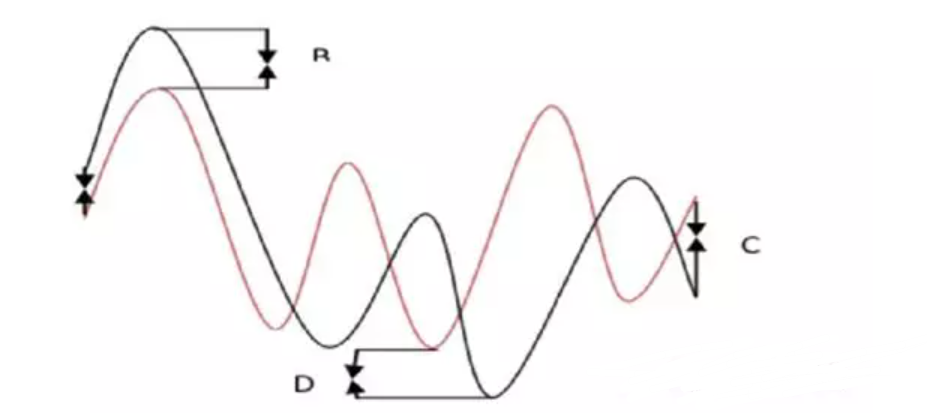如果正常的计算Q和C之间的DTW距离，这样计算量很大，我们可以为Q设置上下界（U和L），然后使用U和L和C进行距离计算（C和U、L之间直接通过对应时间步计算，不用对齐），这个距离属于估算，如果估算出来的这个距离大于设置阈值，我们就认为Q和C之间的差距太大了，二者不匹配。Lower Bounding存在两个算法变种：LB-kim和LB-koegh

LB-kim：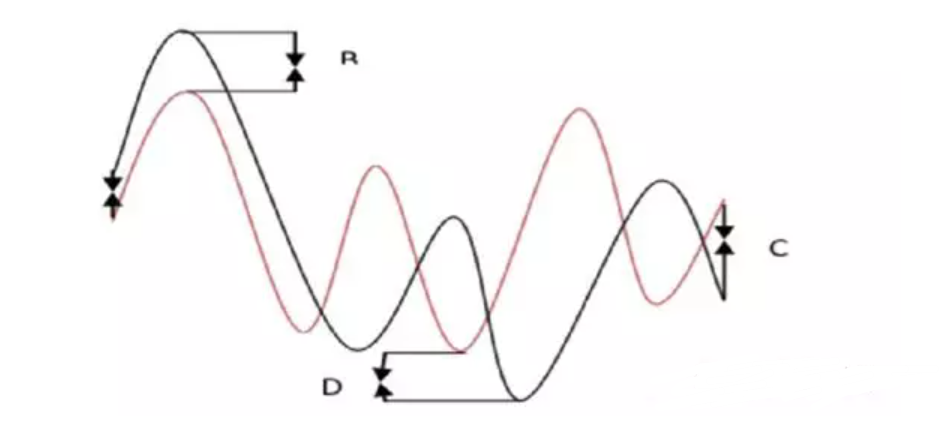直接找到Q和C的四个对应的点，起始点，终点，最高点，最低点，计算这四个点的距离和，如果超过阈值，那么我们就认为这个Q和C不匹配。

LB-koegh：

直接找到Q和C的两个对应的点，最高点和最低点，如果超过阈值我们就认为这个Q和C不匹配。

我们可以看出来这两种方式计算的点比较少，所以计算量极少，速度会很快，但是会有问题，就是不精确，仅仅通过几个点就确定了序列的匹配程度，这样会有误差的。

Early Abandoning of ED and LB_Keogh

这个是将ED和LB进行结合，因为ED计算（DTW）比较精确，但是计算量大，而LB比较粗略，但计算量小，我们将二者结合，如图所示，我们将k=11之前使用DTW计算，K=11之后我们使用LB来计算，此时我们将二者加起来，如果这个超过阈值，我们就可以认为Q和C不匹配

一些新的技巧总结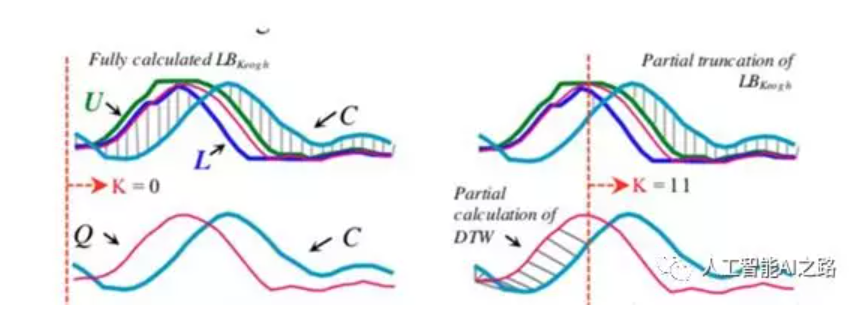Early Abandoning Z-Normalization

我们在使用DTW算法的时候，往往需要对数据进行归一化操作，这样可以提高效率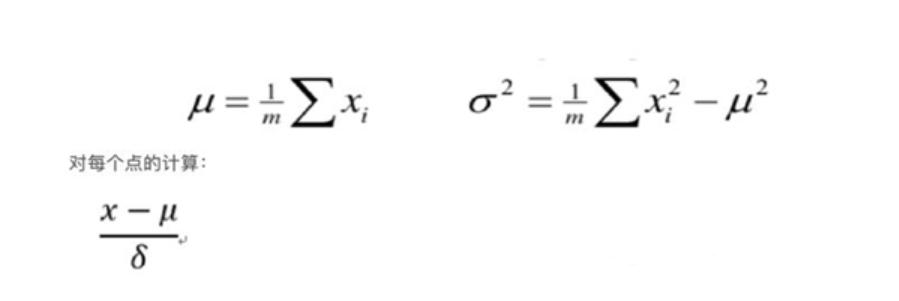归一化操作

那么如果先进行归一化再进行动态规划，这样的问题就是一旦Q和C不匹配，那么就对C白白归一化了，那么我们可以这样的，每标准化一点就对这点进行ED计算，如果计算过程中总距离一旦超过阈值，就立即停止计算，以后的也不用进行归一化了，这样后面的点就不用归一化了，这样计算量就减少了。

Reordering Early Abandoning

DTW计算的时候，一般从序列匹配的起点开始计算，我们发现当计算到第9个时间步的时候，那么就发现它超过了阈值，我们就认为二者是不匹配的，就停止计算了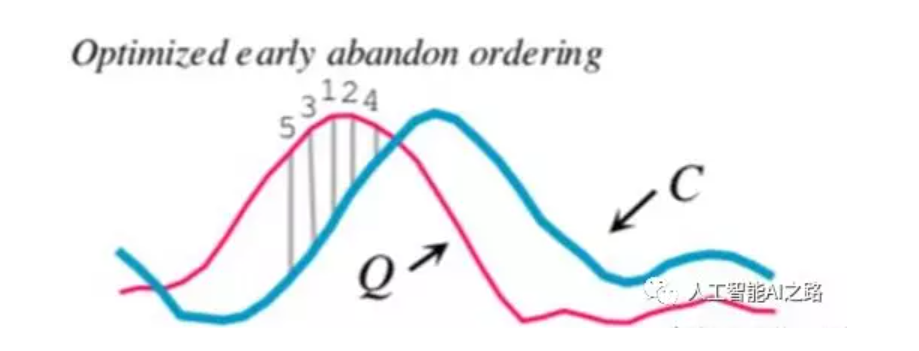现在我们计算的时候不从起点开始计算，比如我们可以从中间的某个特殊的时间步（一般是Q中距离均值0比较远的序列段，用这段来和C进行距离计算，这个序列段再标准化的过程中就可以找到）计算。论文中的reorder early abounding 这部分的做法是对Q序列进行norm处理，然后对所有时间点元素值取绝对值，最后进行降序排列，以此来找到异常点？

这样，我们只计算（直接按照时间步计算距离，估算）了五个时间步就发现，Q和C距离超过阈值，二者是不匹配的，这样就直接停止计算。

以Q为基础的LB和以C为基础的LB我们使用LB的方法都是对Q来使用的，然也可以对C来使用，这样的好处是对Q使用可以过滤掉一部分不匹配的序列，对C使用又可以过滤掉一批不匹配的序列
多种LB方式综合使用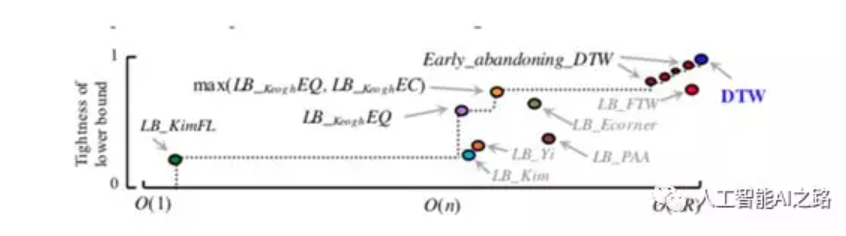我们可以看到横轴表示时间复杂度，纵轴表示可靠性，我们可以认为越靠近左上角的算法是越好的。

总结

我们需要找到与Q距离最短的C，当序列很长和C数量很多的时候，一个很重要的问题就是过滤，也就是及时停止距离计算,上面的技巧就是在做这个工作，通过各种方式，只要发现Q和C的距离计算超过阈值就抛弃C，因为计算Q和C之间的DTW真的很费时间。

参考论文：Searching and Mining Trillions of Time Series Subsequences under Dynamic Time Warping

展开全文人工智能 算法 大数据
• LSTM多变量时间序列预测

千次阅读 多人点赞 2021-03-27 20:40:42多变量时间序列 LSTM keras
• CLUSTAL是一种渐进的比对方法，先将多个序列两两比对构建距离矩阵，反应序列之间两两关系；然后根据距离矩阵计算产生系统进化指导树，对关系密切的序列进行加权；然后从最紧密的两条序列开始，逐步引入临近的序列并...
• 作为一名生物科研狗，在饱受实验折磨的同时，相信大家也都多少会受到一些生信软件的“宠爱”比如需要做序列比对，却不知道该用什么软件，不知道怎么设参数、不懂怎么读结果。今天我们详细地给大家介绍一款必会比对...多序列比对要多久时间
• 本文为大家梳理时间序列聚类相关知识，总结有关时间序列相似性度量的方法，希望能为大家学习更高级的时序模型奠定一些基础。 01 相似性度量 要找到相似的时序曲线，并将其聚为一类，首先，我们需要有方法刻画时间...聚类 算法 机器学习 人工智能 python
• 尽管大多数现有方法考虑了时间序列数据中的多个尺度，但它们假设各种尺度对每个样本都同等重要，因此无法捕捉时间序列的动态时间模式。为此，我们提出了时间感知多尺度循环神经网络 (TAMS-RNNs)，它可以解开不同尺度...人工智能 算法 大数据 python 机器学习
• 上文我们已经知道了什么是时间序列的平稳，也见到了一些平稳时间序列和非平稳的时间序列，那么当我们有一新的时间序列数据时，怎么判断它是否是平稳的呢？时间序列平稳检验方法，可分为三类：图形...可视化 python 机器学习 数据分析 人工智能
• 文| Vachel编辑| Sucie转载：时序人00写在前面时间序列是指将同一统计指标的数值按其发生的时间先后顺序排列而成的数列，其中隐藏着一些过去与未来的关系。时间序列分析试图通过研...协方差 机器学习 人工智能 大数据 编程语言
• 同源性 相似性 一致性

千次阅读 2021-05-10 21:23:45
• 时间序列分析 - 23 DTW (时序相似度度量算法) 上 DTW初探 简介     在时序分析中，DTW(Dynamic Time Warping)是用来检测两个时序相似程度的算法，而这个相似... 这两个序列中的元素按照位置一一计python 算法 时间序列 股票 相似度度量python 人工智能
• 特别是，GRAB 首先通过子序列之间的相似性时间序列划分为一组不重叠的片段。然后，它构建一基于片段的图，并采用图划分方法将片段聚类为状态。对真实世界数据集的大量实验证明了我们的 GRAB 方法的有效性和效率...数据库 大数据 编程语言 python 机器学习
• 在前几次分享中我们知道，很时序算法都依赖完整的时序数据进行建模，许多业务也需要数据保持完整，以更好地进行可视化与分析。然而在真实场景中，由于采集能力或网络传输的原因，时序数据常常会有缺...算法 大数据 python 机器学习 人工智能
• Datawhale干货译者：陈超，北京大学，数据派THU 本文约7500字，建议阅读20+分钟本文介绍了时间序列的定义、特征并结合实例给出了时间序列在Python中评价指标和方法。...可视化 python 机器学习 数据分析 深度学习
• 对于几具有挑战时间序列模型，我们证明了与完整数据集上的MCMC相比，速度最多提高了两数量级，同时产生的偏差可忽略不计。 论文标题：Continuous Time Bayesian Networks with Clocks 论文地址：...机器学习 人工智能 深度学习 python 编程语言
• OK，来简单讲述下自己最近所浏览的一篇论文《constructing ordinal partition transition networks from multivariate time series》中译为《从多元时间序列构造有序划分转换网络》 中英文PDF版资源复杂网络
• 当我们有一新的时间序列数据时，怎么判断它是否是平稳的呢？时间序列平稳检验方法，可分为三类：图形分析方法简单统计方法假设检验方法一、图形分析方法图形分析方法是一种最基本、最简单直接的方法...可视化 python 机器学习 数据分析 人工智能
• 在深度学习的知识宝库中，除了前面文章中介绍的RNN，还有一重要的分支：卷积神经网络（CNN），其广泛应用于视觉，视频等二维或者多维的图像领域。卷积网络具有深度，可并行等多种特性，这种技术...卷积 神经网络 网络 python 机器学习
• 比如，每天某产品的用户数量，每月的销售额，这些数据形成了以一定时间间隔的数据。 通过对这些时间序列的分析，从中发现和揭示现象发展变化的规律，并将这些知识和信息用于预测。比如销售量是上升还是下降，销售...python 数据分析
• 在来自不同领域和具有不同特征的多个公共多元时间序列数据集上评估我们的框架，我们证明它的性能明显优于目前最好的回归和分类方法，即使对于仅包含几百个训练样本的数据集也是如此。鉴于科学和工业中几乎所有领域对...数据挖掘 算法 大数据 编程语言 python
• 时间序列模型概述1.1 时间序列的不同分类1.2 确定性时间序列分析方法概述1.3 三种时间序列模型2.指标平滑ES3.移动平均法4.ACF与PACF5.AR6.MA7.ARMA8.ARIMA8.1 差分 1.时间序列模型概述 时间序列是研究数据随时间变化...数据挖掘 时间序列
• 1、引言 2、流时间序列中基于预测的异常检测 3、异常形状的时间序列 4、多维流的异常检测异常检测
• 2、在Pandas上传和加载时间序列(pandas 是基于 Numpy 构建的含有更高级数据结构和工具的数据分析包，类似于 Numpy 的核心是 ndarray，pandas 也是围绕着 Series 和 DataFrame 两核心数据结构展开的 。)3、如何检验...
• 这个过程会在信号的多个时间窗内反复计算时间滞后互相关。然后我们可以分析每个窗口或者取窗口上的总和，来提供比较两者之间领导者跟随者互动差异的评分。 # 加窗的时间滞后互相关 seconds = 5 fps = 30 no_splits...python 数据分析 数据结构 大数据
• 时间序列预处理

千次阅读 2020-12-19 21:43:55
...时间序列预处理流程图（侵删） 下面来详细介绍每阶段的处理 数据预处理流程图 数据预处理-平稳检验机器学习 人工智能 数据分析
• 简介动态时间规整：(Dynamic Time Warping，DTW)定义：用于比较不同长度的两数组或时间序列之间的相似性或计算两者间的距离。例1：a =[1,2,3],b=[3,2,2...算法 python js 机器学习 人工智能...